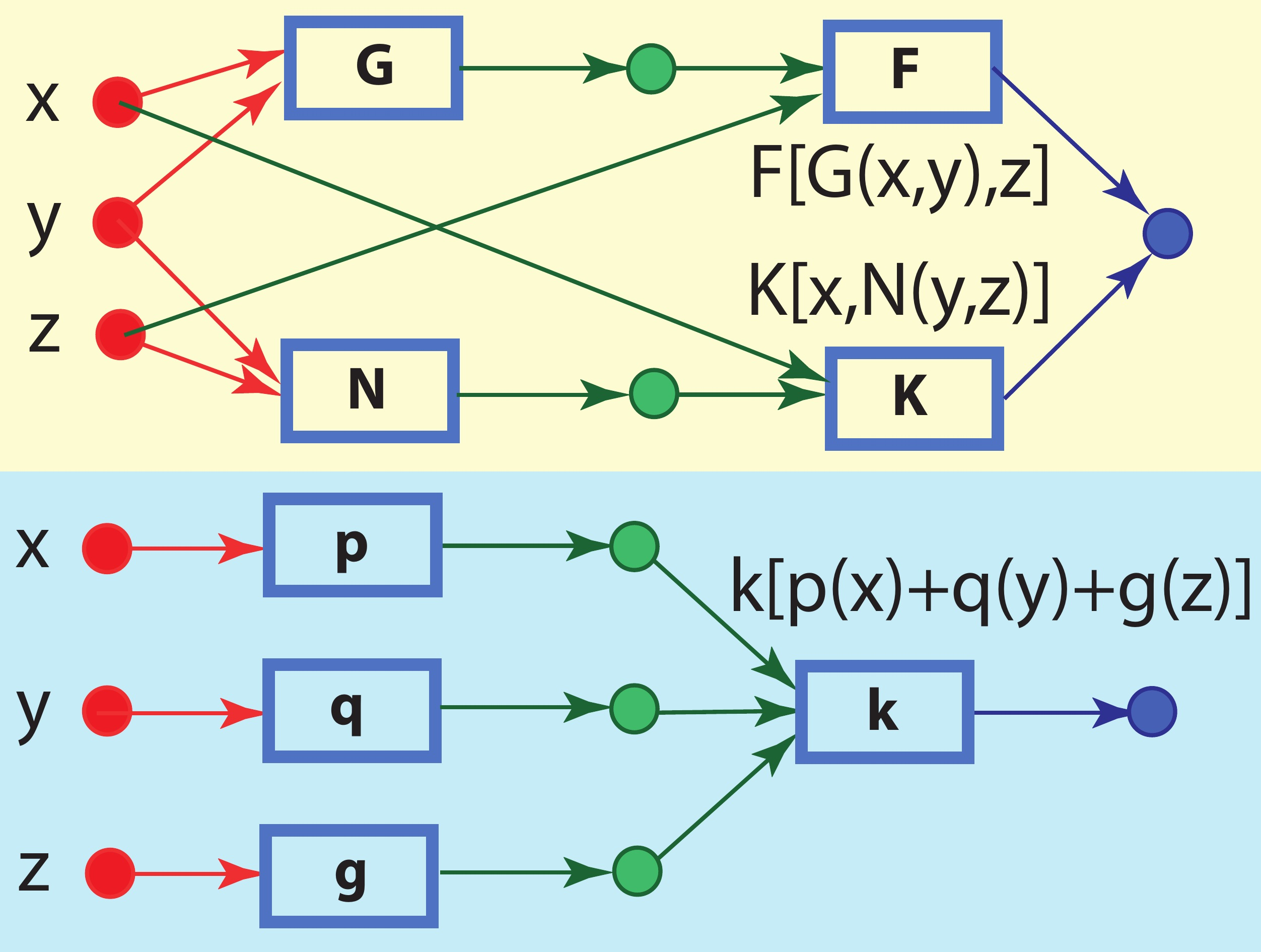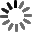El sitio web de la UCLM utiliza cookies propias y de terceros con fines técnicos y de análisis, pero no recaba ni cede datos de carácter personal de los usuarios. Sin embargo, puede haber enlaces a sitios web de terceros, con políticas de cookies distintas a la de la UCLM, que usted podrá aceptar o no cuando acceda a ellos.

Puede obtener más información en la Política de cookies. Aceptar# Functional Equations

Curso de fuentes OpenUCLM

This course introduces functional equations. First we motivate the course using the formulas for the area of a rectangle and a trapezoid, showing that they are not the most correct ones. We give the simple and compound interest formulas together with an interesting interpretation, in terms of account stability. Next, we introduce some classical functional equations, as the associative, the Cauchy's and the Pexider's equations. We continue with the sum of product equations and two examples of applications. Next, we provide a list of ten methods to solve functional equations and illustrate them with some examples. In the final part, we give interesting examples of applications to Economy, Statistics, fatigue, laws of Science and differential equations.

Autores Fecha Enrique Castillo 17/09/2019 Ingles

Me GustaIn this course we introduce functional equations. Some illustrative examples of the real practice are used to present the most common functional equations and other not so common and their general solutions are given. A list of methods to solve functional equations are indicated and explained together with some illustrative examples.

Functional Equations

Enrique Castillo

A course on functional equations
In this lesson we motivate the course of functional equations. We start by deriving the formulas of the areas of a rectangle and a trapezoid, showing that the traditional formulas are not the most convenient. Next, we derive the formula for the sum of the internal angles of a polygon. Finally, we obtain the simple and the compound interst, showing some of their important properties.

In this lesson we provide some classical functional equations. We start with an encryption equation and we continue with the bisymmetry, the associativity and the generalized associativity equations. Since we want the readers to connect these equations with functional networks, we also include some illustrative graphics and expect the reader to enrich from this use of functional equations. Finally, we end with the Cauchy's I, II and III and the Pexider's I, II and III equations. Some examples of application are given.

We provide some classical functional equations, such as an encryption equation, the bisymmetry, the associativity and the generalized associativity equations. We also include some illustrative graphics and expect the reader to connect functional equations with functional networks. Finally, we end with the Cauchy's and the Pexider's equations. Some examples of application are given.

In this lesson we deal with a special functional equation that allow us to build some interesting models. The name comes from the fact that the equation is the sum of products of functions of x and functions of y. In particular, we present the problem of designing a cover of a sports building suvh that the sections parallel to the coordinate axes are second degree polynomials, and the most general bivariate distribution with normal conditionals, that is an important problem. It can be generalized to other conditional families.

In this lesson we deal with a special functional equation that allow us to build some interesting models. In particular, we present the problem of designing a cover of a sports building and the most general bivariate distribution with normal conditionals, that is an important problem.

In this lesson we continue with some examples of practical applications that lead to functional equations. We combine the theory and the practice to make it more enjoyable and to motivate the students."; We start with the translation equation which is motivated with damage accumulation curves that must satisfy this equation. We continue with the Euler formula for polyhedra and iterative methods. Finally, we present some functional equations that arise in expert systems and artificial intelligence and present a model for medical diagnosis.

We give some examples of practical applications that lead to functional equations. We start with the translation equation which is motivated with damage accumulation curves. We continue with the Euler formula for polyhedra and iterative methods. Finally, we present some functional equations that arise in expert systems and artificial intelligence and present a model for medical diagnosis.

In this lesson we describe some methods to solve functional equations. The reader can take the opportunity to learn some techniques that are very useful to solve real life problems. The list of methods include replacing variables by given values, transforming variables, transforming functions, using more general equations, derivation, integration, inductive and iterative methods, separation of variables and mixed methods. Some illustrative examples are given to clarify how the different methods work.

In this lesson we describe some methods to solve functional equations. The reader can take the opportunity to learn some techniques that are very useful to solve real life problems.

This block provides a series of economic problems that can be stated as functional equations, which reproduce important properties to be satisfied. Next, they are solved to obtain the most general models that satisfies the stated conditions. In particular we study some interest and taxation problems and some monopoly and duopoly problems.

Applications to Economy

Enrique Castillo

We present some economic problems modelled by functional equations, such as interest and taxation formulas and monopoly and duopoly models. We invite the reader to initiate a nice trip, which consists of building models based on their properties. Once this system is solved, we obtain the only possible models. No other models satisfy those properties.

Some probability and statistical problems are stated in terms of functional equations and solved. Some examples are the case of Bayesian conjugate distributions, the sum of a random number of random variables, the maximum stability distribution models, etc.

We give some examples of applications of functional equations to probability and Statistics, such as maximum stability, conjugate families, sums of a random number of random variables, reproductivity, etc. Finally, we identify families of bivariate distributions with linear regressions and conditionals in location scale families.

This block presents some fatigue models that are derived using functional equations. First, we give a model based on the independence assumption and later, we give three models assuming possible dependence among the strengths of the small pieces that make the whole longitudinal element. Finally, we discuss the problem of obtaining a consensus model.

Fatigue Models

Enrique Castillo

We present some fatigue models that are derived using functional equations. First, we give a model based on the independence assumption and later, we give three models assuming possible dependence among the strengths of the small pieces that make the whole longitudinal element. Finally, we discuss the problem of obtaining a consensus model.

In this lesson we discuss the validity of physical formulas. We discover the conditions for physical formulas to be valid, that is, invariant with respect to changes of scale and in some cases changes of origin. Functional equatio ns are the ideal tool to state ythis problem.

Laws of Science

Enrique Castillo

We discuss how to build valid formulas relating physical expressions. We show that the usual formulas in physics and engineering cannot have a different structure. We distinguish between fundamental and secondary variables, and also between ratio and interval scale variables. The final conclusion is that we must be careful when building models because we can have serious problems if we do not respect the required and necessary rules.

In this lesson we show how functional equations can be an advantageous alternative to differential equations. We will use a beam example to show how the classical statement of differential equation problems, based on a balance with a differential element, can be replaced with the balance of a finite element, to get the alternative functional equation. We also will try to emphasize the similitudes of the two resulting problems

In this lesson we show how functional equations can be an advantageous alternative to functional equations. A beam example is used to show how the classical statement of differential equation problems, based on a balance with a differential element, can be replaced with the balance of a finite element, to get the alternative functional equation. We also will try to emphasize the similitudes of the two resulting problems.

Here are all the slideshows from Functional Equations

Slideshows

Enrique Castillo